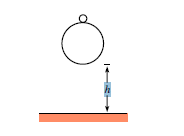# Basketball and tennis ball: Part IA tennis ball with (small) mass m2 sits on top of a basketball with (large) mass m1. The bottom of the basketball is a height h above the ground, and the bottom of the tennis ball is a height h + d above the ground. The balls are dropped. To what height does the tennis ball bounce?

Note: Work in the approximation where m1 is much larger than m2, and assume that the balls bounce elastically.

This is a part of the 'Harvard Problem of the Week' set. Go on and try out the whole bunch of them.

×